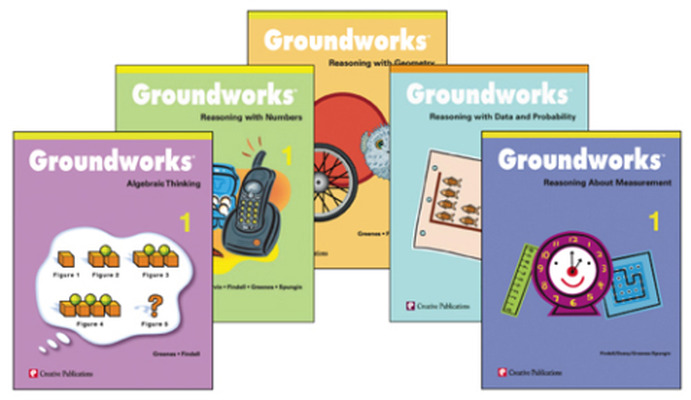Each grade level set includes one book from each of the five Groundworks series:

• Algebra
• Data and Probability
• Geometry
• Measurement
• Number Sense and Skill PracticeText Organization

Each text in Groundworks contains 15 different sets of reproducible problem pages. A set refers to a specific type of mathematical reasoning problem. Each set contains six different problems for ample practice reinforcement. With 15 different sets of six problems, each text totals 90 different mathematical reasoning problems.

Problem Sets

Each problem set consists of eight pages and begins with a teaching information page. This page contains several features to help guide the teacher through the activity, either as a class or individually. Following the teacher page are six pages of student problems, each containing one problem, all addressing the same concept. The final page in the problem set is a solutions page.

## Program Components

•